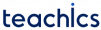# SR flip flop

Flip flops are synchronous bistable devices, also known as bistable multivibrators. The term synchronous means that changes in the output occur in synchronization with control input called a clock.

The basic form of an edge triggered flip-flop is the SR flipflop. The logic diagram of an SR flip-flop is shown in the figure.

## Circuit Diagram of SR Flip flop

The SR flip flop is designed by adding two NAND gates to a basic SR latch. The advantage of this clocked circuit is that the inputs R and S are considered only when the clock pulse is high. The circuit of the SR flip flop using the NAND gate is shown below.

## Working of SR Flip flop

When Clk = 0, irrespective of the value of S or R, the value ofandwill be whatever the values stored in the previous state of the flip flop.

When Clk = 1, the output of the first two NAND gates, followed by inputs S and R, will beand. When S=1, we get SET state and when R=1, we get the RESET state.

As before, the condition R = S = 1 is indeterminate and should be avoided when Clk is also 1.

This operation is described in the following truth table. Hereindicated the present state andindicates the next state. The characteristics table can be obtained from the truth table is given below.0 X X Qn
1 0 0 Qn
1 0 1 0
1 1 0 1
1 1 1 Invalid

Truth table0 0 0 0
0 0 1 0
0 1 0 1
0 1 1 X
1 0 0 1
1 0 1 0
1 1 0 1
1 1 1 X

Characteristics table

From these two tables, we know the transition from the present state to the next state. To find the latch input conditions that will cause the specified transition, We need a table that lists the required inputs for a given change of state. It is called an excitation table.

We can also obtain the expression forfrom the characteristics table using the K-Map technique as follows.0 0 0 X
0 1 1 0
1 0 0 1
1 1 X 0

Excitation Table

Subscribe
Notify of